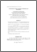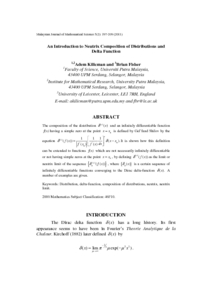# An introduction to neutrix composition of distributions and delta function

## Citation

Kilicman, Adem and Fisher, Brian (2011) An introduction to neutrix composition of distributions and delta function. Malaysian Journal of Mathematical Sciences, 5 (2). pp. 197-209. ISSN 1823-8343; ESSN: 2289-750X

## Abstract

The composition of the distribution g(s) (x) and an infinitely differentiable function f (x) having a simple zero at the point x = x0 is defined by Gel’fand Shilov by the equation g(s) (f (x)). It is shown how this definition can be extended to functions f (x) which are not necessarily infinitely differentiable or not having simple zeros at the point x = x0, by defining g(s) (f (x)) as the limit or neutrix limit of the sequence {g(s)n (f(x))} where {gn (x)} is a certain sequence of infinitely differentiable functions converging to the Dirac delta-function g(x). A number of examples are given.Preview
PDF
38922.pdfView Item